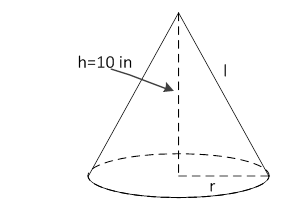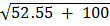### Sample Problem

If the volume of the cone is 550 in3, what is its surface area? Use 3.14 for π and round the answer to the nearest hundredth.The surface area = in2.

#### Solution

V = 1/3 × πr2 × h

r2 = (3 × V) / (π × h) = (3 × 550) / (3.14 × 10) = 52.55 in2

r == 7.25 in

l == 12.35 in

So, SA = πr2 + πrl

= 3.14 × 7.25 × 7.25 + 3.14 × 7.25 × 12.35

= 446.19 in2